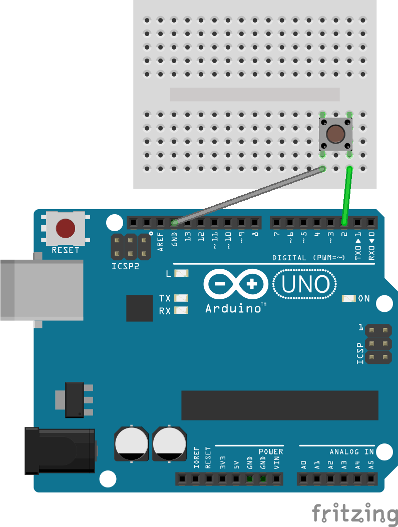# Bare Metal Micro

## AVR Digital I/O

### 5. Inputs

Using digital I/O pins as inputs gives us the ability to do things like read the state of a pushbutton or hall effect sensor. Just like outputs, configuring and using pins as inputs is easy to do.

There are two main steps for using digital inputs:

1. Set the direction of the pin to input
2. Read the value of the pin

To set the direction of the pin to be an input you will use the Data Direction Register. Each port has a data direction register that is used to set the direction of each of its pins. A value of 0 in the data direction register indicates that the pin is an input. At reset all bits in the data direction register are set to 0. The following example shows setting pin PD2 to be an input. Read the Wikipedia page on bit manipulation to get a refresher on clearing bits in C.

To clean things up a bit we can use the _BV macro which converts a bit number into a byte value.

To read the value of a pin you will use the Port Pin Input Register. Each port has a pin input register that is used to read the value of each of its pins. A value of 1 in the port pin input register indicates a high value on the pin. A value of 0 in the port pin input register indicates a low value on the pin. The following example shows reading the PD2 pin.

In general, its a bad idea to leave input pins floating. Instead, pull-up or pull-down resistors should be used so that pins are always in a well defined logic state. The good news is that the AVR has builtin pull-up resistors that you can use, reducing the number of external components. To enable the pull-up resistor on a pin, you will use the Data Register. A value of 1 in the port data register enables the pull-up resistor on the pin. A value of 0 in the port data register disables the pull-up resistor on the pin. The following example show enabling the pull-up resistor on the PD2 pin.

A full working example of applying what we have covered so far is listed below. This example configures PD2 as an input with a pull-up resistor and then repeatedly checks the pin. If the pin is high, PB5 outputs a low value, which will turn the LED off. If the pin is low, PB5 outputs a high value, which will turn the LED on.

The circuit for this example should be wired according to Figure 1. If you run this example on an Arduino Uno, you will see the LED that is connected to Arduino pin 13 turning ON when the push button is pressed and turning OFF when the push button is released.Figure 1. Input example wiring

Make sure to orient the push button correctly. If it is rotated the wrong way, the LED will always be on.